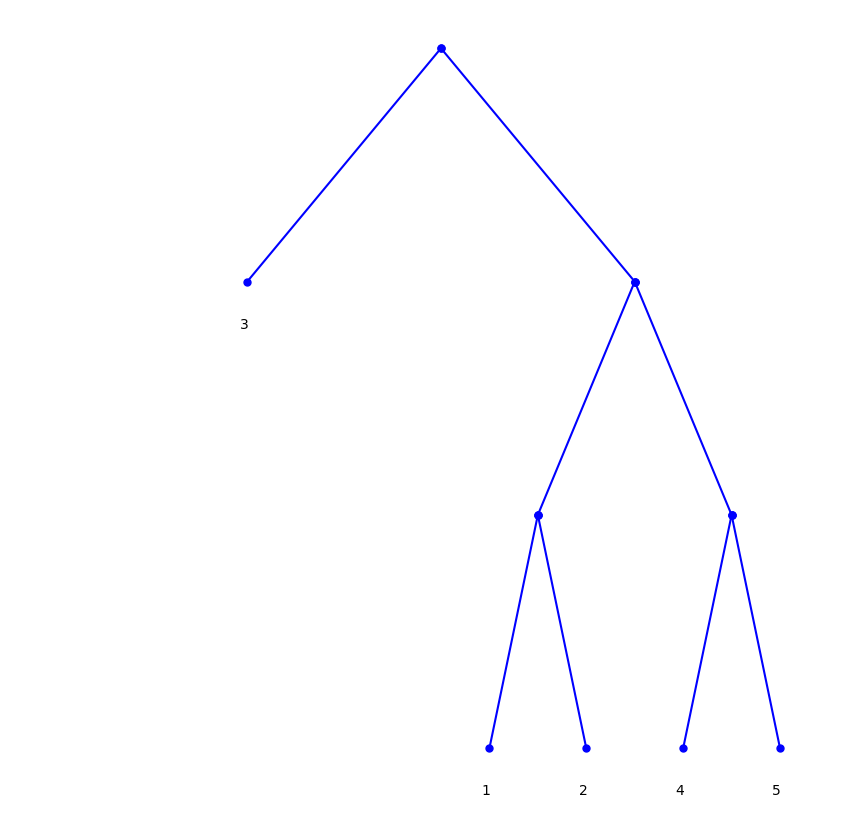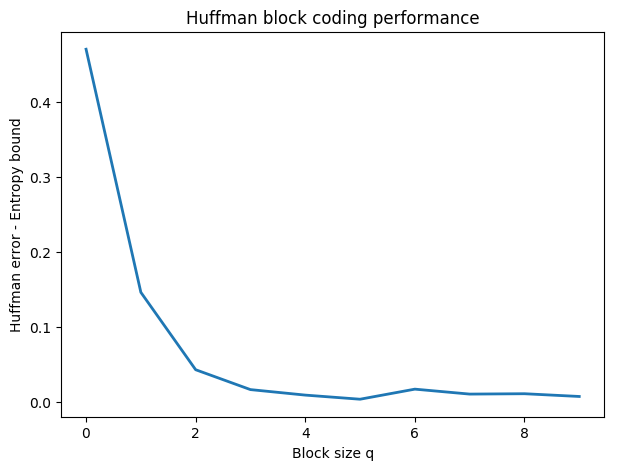# Entropic Coding and Compression¶

Important: Please read the installation page for details about how to install the toolboxes. $\newcommand{\dotp}{\langle #1, #2 \rangle}$ $\newcommand{\enscond}{\lbrace #1, #2 \rbrace}$ $\newcommand{\pd}{ \frac{ \partial #1}{\partial #2} }$ $\newcommand{\umin}{\underset{#1}{\min}\;}$ $\newcommand{\umax}{\underset{#1}{\max}\;}$ $\newcommand{\umin}{\underset{#1}{\min}\;}$ $\newcommand{\uargmin}{\underset{#1}{argmin}\;}$ $\newcommand{\norm}{\|#1\|}$ $\newcommand{\abs}{\left|#1\right|}$ $\newcommand{\choice}{ \left\{ \begin{array}{l} #1 \end{array} \right. }$ $\newcommand{\pa}{\left(#1\right)}$ $\newcommand{\diag}{{diag}\left( #1 \right)}$ $\newcommand{\qandq}{\quad\text{and}\quad}$ $\newcommand{\qwhereq}{\quad\text{where}\quad}$ $\newcommand{\qifq}{ \quad \text{if} \quad }$ $\newcommand{\qarrq}{ \quad \Longrightarrow \quad }$ $\newcommand{\ZZ}{\mathbb{Z}}$ $\newcommand{\CC}{\mathbb{C}}$ $\newcommand{\RR}{\mathbb{R}}$ $\newcommand{\EE}{\mathbb{E}}$ $\newcommand{\Zz}{\mathcal{Z}}$ $\newcommand{\Ww}{\mathcal{W}}$ $\newcommand{\Vv}{\mathcal{V}}$ $\newcommand{\Nn}{\mathcal{N}}$ $\newcommand{\NN}{\mathcal{N}}$ $\newcommand{\Hh}{\mathcal{H}}$ $\newcommand{\Bb}{\mathcal{B}}$ $\newcommand{\Ee}{\mathcal{E}}$ $\newcommand{\Cc}{\mathcal{C}}$ $\newcommand{\Gg}{\mathcal{G}}$ $\newcommand{\Ss}{\mathcal{S}}$ $\newcommand{\Pp}{\mathcal{P}}$ $\newcommand{\Ff}{\mathcal{F}}$ $\newcommand{\Xx}{\mathcal{X}}$ $\newcommand{\Mm}{\mathcal{M}}$ $\newcommand{\Ii}{\mathcal{I}}$ $\newcommand{\Dd}{\mathcal{D}}$ $\newcommand{\Ll}{\mathcal{L}}$ $\newcommand{\Tt}{\mathcal{T}}$ $\newcommand{\si}{\sigma}$ $\newcommand{\al}{\alpha}$ $\newcommand{\la}{\lambda}$ $\newcommand{\ga}{\gamma}$ $\newcommand{\Ga}{\Gamma}$ $\newcommand{\La}{\Lambda}$ $\newcommand{\si}{\sigma}$ $\newcommand{\Si}{\Sigma}$ $\newcommand{\be}{\beta}$ $\newcommand{\de}{\delta}$ $\newcommand{\De}{\Delta}$ $\newcommand{\phi}{\varphi}$ $\newcommand{\th}{\theta}$ $\newcommand{\om}{\omega}$ $\newcommand{\Om}{\Omega}$

This numerical tour studies source coding using entropic coders (Huffman and arithmetic).

In :
using PyPlot
using NtToolBox


## Source Coding and Entropy¶

Entropic coding converts a vector $x$ of integers into a binary stream $y$. Entropic coding exploits the redundancies in the statistical distribution of the entries of $x$ to reduce as much as possible the size of $y$. The lower bound for the number of bits $p$ of $y$ is the Shannon bound :

$$p=-\sum_ih(i)\log_2(h(i))$$

where $h(i)$ is the probability of apparition of symbol $i$ in $x$.

Fist we generate a simple binary signal $x$ so that $0$ has a probability $p$ to appear in $x$.

Probability of 0.

In :
p = 0.1;


Size.

In :
n = 512;


Signal, should be with token 1,2.

In :
x = (rand(n) .> p) + 1;


One can check the probabilities by computing the empirical histogram.

In :
h = [sum(x .== 1); sum(x .== 2)]
h = h/sum(h)

print("Empirical p = $(h)")  Empirical p = 0.109375 We can compute the entropy of the distribution represented as a vector$h$of proability that should sum to 1. We take a max to avoid problems with null probabilties. In : e = - sum(h.*log2([max(e,1e-20) for e in h])) print("Entropy =$e")

Entropy = 0.4980278865344765

## Huffman Coding¶

A Hufman code $C$ associates to each symbol $i$ in $\{1,...,m\}$ a binary code $C_i$ whose length is as close as possible to the optimal bound $-\log_2\left(h(i)\right)$, where $h(i)$ is the probability of apparition of the symbol $i$.

We select a set of proabilities.

In :
h = [.1, .15, .4, .15, .2];


The tree $T$ contains the codes and is generated by an iterative algorithm. The initial "tree" is a collection of empty trees, pointing to the symbols numbers.

In :
m = length(h)
T=Array{Any,1}(zeros(m)); # create an empty tree


We build iteratively the Huffman tree by grouping together the two erees that have the smallest probabilities. The merged tree has a probability which is the sum of the two selected probabilities.

Initial probability.

In :
#we use the symbols i = 0,1,2,3,4 (as strings) with the associated probabilities h(i)

for i in 1:m
T[i] = (h[i],string(i))
end


Iterative merging of the leading probabilities.

In :
while length(T) > 1

sort!(T) #sort according to the first values of the tuples (the probabilities)
t = tuple(T[1:2]...)
q = T + T
T = [T[3:end]; [(q,t)]]
end


We trim the computed tree by removing the probabilities.

In :
function trim(T)
T0 = T
if typeof(T0) == String
return T0
else
return (trim(T0),trim(T0))
end
end
T = trim(T);


We display the computed tree.

In :
plot_hufftree(T);Once the tree $T$ is computed, one can compute the code $C_{i}$ associated to each symbol $i$. This requires to perform a deep first search in the tree and stop at each node.

In :
codes = Dict()

function huffman_gencode(T,codes,c)
if typeof(T) == String #test if T is a leaf
codes[T] = c
else
huffman_gencode(T,codes, string(c, "0"))
huffman_gencode(T,codes, string(c, "1"))
end
end

huffman_gencode(T,codes,"") ;


Display the code.

In :
for e in keys(codes)
println(string("Code of token ", e, ": ", codes[e]))
end

Code of token 4: 110
Code of token 1: 100
Code of token 5: 111
Code of token 2: 101
Code of token 3: 0


We draw a vector $x$ according to the distribution $h$.

Size of the signal.

In :
n = 1024;


Randomization.

In :
x = rand_discr(h, m=n);


Exercise 1

Implement the coding of the vector $x$ to obtain a binary vector $y$, which corresponds to replacing each sybmol $x(i)$ by the code $C_{x(i)}$.

In :
include("NtSolutions/coding_2_entropic/exo1.jl")

In :
## Insert your code here.


Compare the length of the code with the entropy bound.

In :
e = - sum(h.*log2([max(e,1e-20) for e in h]))
println("Entropy bound = $(n*e)") println("Huffman code =$(length(y))")

Entropy bound = 2197.9538889431196
Huffman code  = 2212


Decoding is more complicated, since it requires to iteratively parse the tree $T$.

Initial empty decoded stream.

In :
x1 = [];


Perform decoding.

In :
T0 = T
for e in y
if e == '0'
T0 = T0
else
T0 = T0
end
if typeof(T0) == String
append!(x1,T0)
T0 = T
end
end


We test if the decoding is correct.

In :
err = norm(x-map(x->parse(Int, x), x1))
print("Error (should be zero) : $err")  Error (should be zero) : 0.0 ## Huffman Block Coding¶ A Huffman coder is inefficient because it can distribute only an integer number of bit per symbol. In particular, distribution where one of the symbol has a large probability are not well coded using a Huffman code. This can be aleviated by replacing the set of$m$symbols by$m^q$symbols obtained by packing the symbols by blocks of$q$(here we use$m=2$for a binary alphabet). This breaks symbols with large probability into many symbols with smaller proablity, thus approaching the Shannon entropy bound. Generate a binary vector with a high probability of having 1, so that the Huffman code is not very efficient (far from Shanon bound). Proability of having 0. In : t = .12;  Probability distriution. In : h = [t, 1-t];  Generate signal. In : n = 4096*2 x = (rand(n) .> t) + 1;  For block of length$q=3$, create a new vector by coding each block with an integer in$\{1,...,m^q=2^3\}$. The new length of the vector is$n_1/q$where$n_1=\lceil n/q\rceil q$. Block size. In : q = 3;  Maximum token value. In : m = 2;  New size. In : n1 = Int(ceil(n/q)*q);  New vector. In : x1 = zeros(n1) x1[1:length(x)] = x x1[length(x)+1:end] = 1 x1 = x1 - 1 x2 = [] mult = [m^j for j in 0:q-1] for i in 1:q:n1-1 append!(x2,sum(x1[i:i+q-1].*mult)+1) end  In : H = h for i in 1:q-1 Hold = H H = [] for j in 1:length(h) append!(H,[e*h[j] for e in Hold]) end end  A simpler way to compute this block-histogram is to use the Kronecker product. In : H = h for i in 1:q-1 H = kron(H, h) end  Exercise 2 For various values of block size$k\$, Perform the Huffman coding and compute the length of the code. Compare with the entropy lower bound.

In :
include("NtSolutions/coding_2_entropic/exo2.jl");

Entropy bound = 0.5293608652873644
---
Huffman(block size = 1) = 1.0
Huffman(block size = 2) = 0.676025390625
Huffman(block size = 3) = 0.5728759765625Huffman(block size = 4) = 0.54638671875
Huffman(block size = 5) = 0.5390625
Huffman(block size = 6) = 0.5335693359375
Huffman(block size = 7) = 0.5469970703125
Huffman(block size = 8) = 0.5404052734375
Huffman(block size = 9) = 0.5408935546875
Huffman(block size = 10) = 0.5372314453125

In :
## Insert your code here.